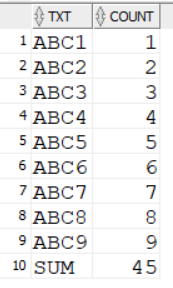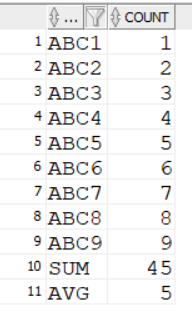## total and average - simultaneously in the summary

Hi everyone,
Such a simple way to do the sum of the values of the selected columns, or the average and others. But how can you make the summary be composed of two rows, in the first SUM, and in the second row, for example, the average?

``````with x as (
select level as lvl, 'ABC'||level as txt
from dual
connect by level < 10
)
select
case when grouping(txt) > 0 then 'SUM' else txt end as txt,
sum(lvl) as count
from x
group by rollup ((txt, lvl))
``````I would like something like this:without repeating the query and using a union all solution....
thx.

This post has been answered by Solomon Yakobson on Sep 5 2022